/

### Extreme Events – Specimen Question A.7.3

Suppose that the regimes in A.7.2 have the following distributional characteristics and transition probabilities:

Distributional characteristics

 Regime 1 Regime 2 Means Covariances Means Covariances Asset A B A B A 0.03 0.01 0.005 0.01 0.02 0.02 B 0.06 0.005 0.02 -0.01 0.02 0.03

Transition probabilities

 State at start of next period State at start of this period Regime 1 Regime 2 Regime 1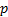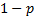Regime 2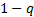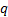where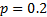and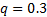.

(a)    What are the conditional means and covariance matrices of the distributions for the next period if the world is in (i) Regime 1, (ii) Regime 2?

(b)   What in broad terms is the impact on optimal portfolios of increasingandby equal amounts, i.e. the likelihood that we switch states over the coming period whatever the state of the world we are currently in?